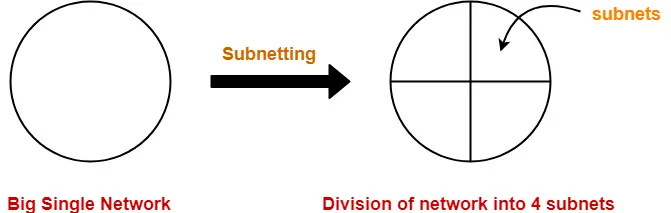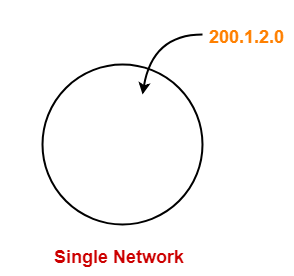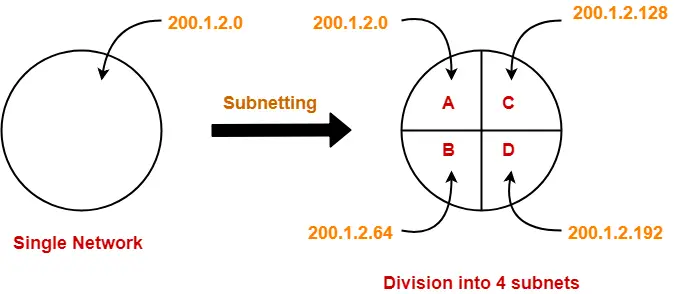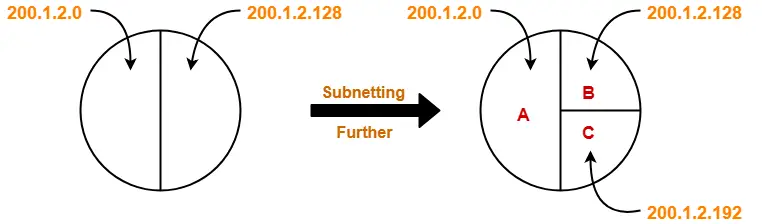# Subnet Mask | How to Calculate Subnet Mask

## Subnetting in Networking-

Before you go through this article, make sure that you have gone through the previous article on Subnetting.

We have discussed-

• Subnetting is a process of dividing a single network into multiple sub networks.
• The number of sub networks created depends upon the requirements.Subnet mask is a 32 bit number which is a sequence of 1’s followed by a sequence of 0’s where-

• 1’s represent the global network ID part and the subnet ID part.
• 0’s represent the host ID part.

## How to Calculate Subnet Mask?

For any given IP Address, the subnet mask is calculated-

• By setting all the bits reserved for network ID part and subnet ID part to 1.
• By setting all the bits reserved for host ID part to 0.

## Subnet Mask Examples-

Now, let us discuss some examples on how to calculate subnet mask for any given network-

## Example-01:

Consider we have a network having IP Address 200.1.2.0.Clearly, this IP Address belongs to class C.

In class C-

• 24 bits are reserved for the Network ID part.
• 8 bits are reserved for the Host ID part.

Subnet mask is obtained-

• By setting the first 24 bits to 1.
• By setting the remaining 8 bits to 0.

= 11111111.11111111.11111111.00000000

= 255.255.255.0

## Example-02:

Consider a single network having IP Address 200.1.2.0 is divided into 4 subnets as shown-Now, let us calculate the mask subnet for each subnet.

For each subnet-

• 24 bits identify the global network.
• 2 bits identify the subnet.
• 6 bits identify the host.

For each subnet, subnet mask is obtained-

• By setting the first 26 bits to 1.
• By setting the remaining 6 bits to 0.

= 11111111.11111111.11111111.11000000

= 255.255.255.192

### NOTE

In fixed length subnetting,

All the subnets have same subnet mask since the size of each subnet is same.

## Example-03:

Consider a single network having IP Address 200.1.2.0 is divided into 3 subnets as shown-Now, let us calculate the subnet mask for each subnet.

### For Subnet A-

For subnet A-

• 24 bits identify the global network.
• 1 bit identify the subnet.
• 7 bits identify the host.

For subnet A, subnet mask is obtained-

• By setting the first 25 bits to 1.
• By setting the remaining 7 bits to 0.

= 11111111.11111111.11111111.10000000

= 255.255.255.128

### For Subnet B And Subnet C-

For subnet B and subnet C-

• 24 bits identify the global network.
• 2 bits identify the subnet.
• 6 bits identify the host.

For subnet B and subnet C, subnet mask is obtained-

• By setting the first 26 bits to 1.
• By setting the remaining 6 bits to 0.

= 11111111.11111111.11111111.11000000

= 255.255.255.192

### NOTE

In variable length subnetting,

All the subnets do not have same subnet mask since the size of each subnet is not same.

## Use of Subnet Mask-

• Subnet mask is used to determine to which subnet the given IP Address belongs to.
• To know more, Read here.

## Note-01:

Default mask for different classes of IP Address are-

• Default subnet mask for Class A = 255.0.0.0
• Default subnet mask for Class B = 255.255.0.0
• Default subnet mask for Class C = 255.255.255.0

## Note-02:

• Network size is the total number of hosts present in it.
• Networks of same size always have the same subnet mask.
• Networks of different size always have the different subnet mask.

## Note-03:

• For a network having larger size, its subnet mask will be smaller (number of 1’s will be less).
• For a network having smaller size, its subnet mask will be larger (number of 1’s will be more).

## Problem-01:

If the subnet mask 255.255.255.128 belongs to class C, find-

1. Number of subnets
2. Number of hosts in each subnet

## Solution-

= 255.255.255.128

= 11111111.11111111.11111111.10000000

Since 25 bits contain the value 1 and 7 bits contain the value 0, so-

• Number of Net ID bits + Number of Subnet ID bits = 25
• Number of Host ID bits = 7

Now,

• It is given that subnet mask belongs to class C.
• So, Number of Net ID bits = 24.

Substituting in the above equation, we get-

Number of Subnet ID bits

= 25 – 24

= 1

Thus,

 Number of subnets = 21 = 2

Since number of Host ID bits = 7, so-

 Number of hosts per subnet = 27 – 2 = 126

## Problem-02:

If a class B network has a subnet mask of 255.255.248.0, what is the maximum number of hosts per subnet?

1. 1022
2. 1023
3. 2046
4. 2047

## Solution-

= 255.255.248.0

= 11111111.11111111.11111000.00000000

Since 21 bits contain the value 1 and 11 bits contain the value 0, so-

• Number of Net ID bits + Number of Subnet ID bits = 21
• Number of Host ID bits = 11

Since number of Host ID bits = 11, so-

 Number of hosts per subnet = 211 – 2 = 2046

Thus, Option (C) is correct.

To gain better understanding about Subnet Mask,

Watch this Video Lecture

Next Article- Practice Problems On Subnet Mask

Get more notes and other study material of Computer Networks.

Watch video lectures by visiting our YouTube channel LearnVidFun.

SummaryArticle Name
Subnet Mask | How to Calculate Subnet Mask
Description
What is Subnet Mask? Subnet Mask is a 32 bit number that identifies the subnet to which an IP Address belongs. How to calculate Subnet Mask? Subnet Mask for IP Address is calculated by setting all the bits reserved for network ID and subnet ID to 1 and all the bits reserved for Host ID to 0.
Author
Publisher Name
Gate Vidyalay
Publisher Logo